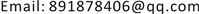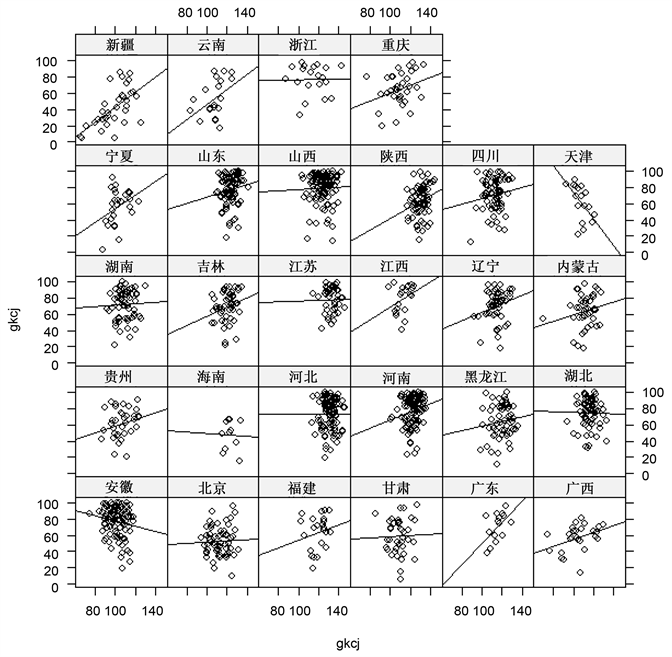﻿ 高数成绩与高考成绩的分层回归模型影响分析 The Analysis on Influence Factors of the Transcripts of Mathematics and Advanced Mathematics Based on Hierarchical Linear Models

Statistics and Application
Vol.06 No.04(2017), Article ID:22403,10 pages
10.12677/SA.2017.64044

The Analysis on Influence Factors of the Transcripts of Mathematics and Advanced Mathematics Based on Hierarchical Linear Models

Chao Qian, Sen Lin, Tong Xue, Xiaoqiang Gao, Kai Zhang

China University of Mining & Technology, BeijingReceived: Oct. 1st, 2017; accepted: Oct. 15th, 2017; published: Oct. 20th, 2017ABSTRACT

Transcript of Mathematics in college entrance examination represents an individual’s learning situation in high school. At the same time, transcript of Advanced Mathematics represents an individual’s learning situation in college. By using hierarchical linear models, this article identifies the relationship between the transcripts of Mathematics and Advanced Mathematics along with some factors that affects student’s Advanced Mathematics’ level greatly. Some suggestions that aimed at increasing college students’ Mathematics level are also being provided. Students come from different provinces and situations, especially educational situations vary in provinces. Taking students’ individual differences in consideration as well, it is obvious that the data has been studied nested structure, which indicates that traditional Hierarchical linear models are not capable of analyzing this category of hierarchical models. The hierarchical models adopted in this article have fully considered both provincial and individual differences in order to establish statistical models properly. The method has overcome the traditional linear models’ limitation on analyzing nested or hierarchical structure. It establishes appropriate hypothetical relationships both inside and among the provinces, and estimate variables from different hierarchy. As a result, this model is more similar to basic phenomenon in reality and its model interpretation is more reasonable.

Keywords:Transcript of Mathematics in College Entrance Examination, Transcripts of Advanced Mathematics, Hierarchical Linear Models1. 引言

2. 模型的介绍

${y}_{ij}={\beta }_{0j}+\sum _{k=1}^{K}{\alpha }_{k}{x}_{kij}+\sum _{l=1}^{L}{\beta }_{lj}{z}_{lij}+{e}_{ij}$ (2.1)

${\beta }_{0j}={\gamma }_{00}+\sum _{h=1}^{H}{\gamma }_{0h}{\omega }_{hj}+{u}_{0j}$ (2.2)

${\beta }_{1j}={\gamma }_{10}+\sum _{h=1}^{H}{\gamma }_{1h}{\omega }_{hj}+{u}_{1j}$ (2.3)

$...$

${\beta }_{Lj}={\gamma }_{L0}+\sum _{h=1}^{H}{\gamma }_{Lh}{\omega }_{hj}+{u}_{Lj}$ (2.4)

${y}_{ij}={\gamma }_{00}+\sum _{h=1}^{H}{\gamma }_{0h}{\omega }_{hj}+\sum _{k=1}^{K}{\alpha }_{k}{x}_{kij}+\sum _{l=1}^{L}{\gamma }_{l0}{z}_{lij}+\sum _{l=1}^{L}\sum _{h=1}^{H}{\gamma }_{lh}{\omega }_{hj}{z}_{lij}+\left({u}_{0j}+\sum _{l=1}^{L}{z}_{lij}{u}_{lj}+{e}_{ij}\right)$ (2.5)

${e}_{ij}~N\left(0,{\sigma }^{2}\right)$ (2.6)

$\left[\begin{array}{l}{u}_{0j}\\ {u}_{1j}\end{array}\right]~N\left[\left(\begin{array}{l}0\\ 0\end{array}\right)\left(\begin{array}{cc}{u}_{{u}_{0}}^{2}& {u}_{{u}_{01}}^{2}\\ {u}_{{u}_{01}}^{2}& {u}_{{u}_{01}}^{2}\end{array}\right)\right]$ (2.7)

$Cov\left({e}_{ij},{u}_{0j}\right)=0,\text{\hspace{0.17em}}Cov\left({e}_{ij},{u}_{1j}\right)=0$ (2.8)

$Cov\left({e}_{ij},{u}_{0j}\right)=0$$Cov\left({e}_{ij},{u}_{1j}\right)=0$

3. 大学生高等数学成绩影响因素建模分析Table 1. The information on freshmen in science and engineering college come from different major and classesFigure 1. Diagram of regression analysis on the transcripts of Mathematics and Advanced Mathematics

1) 模型1：零模型

$\begin{array}{l}\left\{\begin{array}{l}{Y}_{ij}={\beta }_{0j}+{\epsilon }_{ij}\text{}{\epsilon }_{ij}~N\left(0,{\sigma }^{2}\right)\\ {\beta }_{0j}={\gamma }_{00}+{u}_{0j}\text{}{u}_{0j}~N\left(0,{\sigma }_{00}^{2}\right)\end{array}\\ {Y}_{ij}={\gamma }_{00}+{u}_{oj}+{\epsilon }_{ij}\end{array}$

${Y}_{ij}$ ：第j个地区的第i个学生高数成绩

${u}_{0j}$ ：每个地区高数成绩均值与总均值 ${\gamma }_{00}$ 之间的差异；组间差异

${\epsilon }_{ij}$ ：每个学生高数成绩与地区均值之间的差异；组内差异

2) 模型2：用省级别变量解释省际之间变异

$\begin{array}{l}\left\{\begin{array}{l}{Y}_{ij}={\beta }_{0j}+{\epsilon }_{ij}\text{}\text{\hspace{0.17em}}\text{\hspace{0.17em}}\text{\hspace{0.17em}}\text{\hspace{0.17em}}{\epsilon }_{ij}~N\left(0,{\sigma }^{2}\right)\\ {\beta }_{0j}={\gamma }_{00}+{\gamma }_{01}{X}_{4j}+{\gamma }_{02}{X}_{5j}+{\gamma }_{03}{X}_{6j}+{\gamma }_{04}{X}_{7j}+{u}_{0j}\text{}{u}_{0j}~N\left(0,{\sigma }_{00}^{2}\right)\end{array}\\ {Y}_{ij}={\gamma }_{00}+{\gamma }_{01}{X}_{4j}+{\gamma }_{02}{X}_{5j}+{\gamma }_{03}{X}_{6j}+{\gamma }_{04}{X}_{7j}+{u}_{0j}+{\epsilon }_{ij}\end{array}$

${X}_{4}$ ”人均GDP“ ${X}_{5}$ ”人均可支配收入

${X}_{6}$ ”教育投入“ ${X}_{7}$ ”学生教师比

$\left\{\begin{array}{l}{Y}_{ij}={\beta }_{0j}+{\epsilon }_{ij}\\ {\beta }_{0j}={\gamma }_{00}+{\gamma }_{01}{X}_{6j}+{u}_{0j}\end{array}⇒{Y}_{ij}={\gamma }_{00}+{\gamma }_{01}{X}_{6j}+{u}_{0j}+{\epsilon }_{ij}\text{}\left\{\begin{array}{l}{\epsilon }_{ij}~N\left(0,{\sigma }^{2}\right)\\ {u}_{0j}~N\left(0,{\sigma }_{00}^{2}\right)\end{array}$

$ICC=\frac{{\stackrel{^}{\sigma }}_{{u}_{0}}^{2}}{{\stackrel{^}{\sigma }}_{{u}_{0}}^{2}+{\stackrel{^}{\sigma }}^{2}}=\frac{57.27}{57.27+352.98}\approx 0.1396$

$\left(\text{RB}:省级别可解释变异\text{%}\right)=1-\frac{57.27}{89.17}\approx 0.36$

$\left(空模型{\stackrel{^}{\sigma }}_{水平\text{2-}总}^{\text{2}}\right)=89.17+\frac{353.00}{48}\approx 96.52$

$\left(设定模型{\stackrel{^}{\sigma }}_{水平\text{2-}总}^{\text{2}}\right)=57.27+\frac{352.98}{48}\approx 64.62$

$\left(\text{SB}:省级别可解释变异\text{%}\right)=1-\frac{64.62}{96.52}\approx 0.33$

3) 模型3：个体解释变量纳入模型

$\begin{array}{l}\left\{\begin{array}{l}{Y}_{ij}={\beta }_{0j}+{\beta }_{1j}{X}_{1}+{\beta }_{2j}{X}_{2}+{\beta }_{3j}{X}_{3}+{\epsilon }_{ij}\text{}{\epsilon }_{ij}~N\left(0,{\sigma }^{2}\right)\\ {\beta }_{0j}={\gamma }_{00}+{\gamma }_{01}{X}_{6j}+{u}_{0j}\text{}{u}_{0j}~N\left(0,{\sigma }_{00}^{2}\right)\end{array}\\ {Y}_{ij}={\gamma }_{00}+{\gamma }_{01}{X}_{6j}+{\beta }_{1j}{X}_{1}+{\beta }_{2j}{X}_{2}+{\beta }_{3j}{X}_{3}+{u}_{0j}+{\epsilon }_{ij}\end{array}$

${X}_{1}$ ”性别“ ${X}_{2}$ ”年龄“ ${X}_{8}$ ”高考成绩依地区进行中心化

$\left\{\begin{array}{l}{Y}_{ij}={\beta }_{0j}+{\beta }_{1j}{X}_{2}+{\beta }_{2j}{X}_{8}+{\epsilon }_{ij}\\ {\beta }_{0j}={\gamma }_{00}+{\gamma }_{01}{X}_{6j}+{u}_{0j}\end{array}$ $⇒{Y}_{ij}={\gamma }_{00}+{\gamma }_{01}{X}_{6j}+{\beta }_{1j}{X}_{2}+{\beta }_{2j}{X}_{8}+{u}_{0j}+{\epsilon }_{ij}\text{}\left\{\begin{array}{l}{\epsilon }_{ij}~N\left(0,{\sigma }^{2}\right)\\ {u}_{0j}~N\left(0,{\sigma }_{00}^{2}\right)\end{array}$

$\begin{array}{l}\left(\text{RB}:个体可解释变异\text{%}\right)=1-\frac{{\stackrel{^}{\sigma }}^{2}\left(设定模型\right)}{{\stackrel{^}{\sigma }}^{2}\left(空模型\right)}=1-\frac{338.25}{353.00}\approx 0.0418\\ \left(\text{RB}:省可解释变异\text{%}\right)=1-\frac{{\stackrel{^}{\sigma }}^{2}\left(设定模型\right)}{{\stackrel{^}{\sigma }}^{2}\left(空模型\right)}=1-\frac{59.22}{96.52}\approx 0.3864\end{array}$

$\begin{array}{l}\left(\text{SB}:个体可解释变异\text{%}\right)=1-\frac{{\sigma }_{水平\text{1-}总}^{2}\left(设定模型\right)}{{\sigma }_{水平\text{1-}总}^{2}\left(空模型\right)}=1-\frac{338.25+59.22}{353.00+96.52}\approx 0.1158\\ \left(\text{SB}:省可解释变异\text{%}\right)=1-\frac{{\sigma }_{水平\text{2-}总}^{2}\left(设定模型\right)}{{\sigma }_{水平\text{2-}总}^{2}\left(空模型\right)}=1-\frac{59.22+338.25/48}{96.52+353.00/48}\approx 0.3620\end{array}$

4) 模型4：个体解释变量斜率的随机性检验

$\begin{array}{l}\left\{\begin{array}{l}{Y}_{ij}={\beta }_{0j}+{\beta }_{1j}{X}_{8}+{\beta }_{2j}{X}_{1}+{\epsilon }_{ij}\text{}{\epsilon }_{ij}~N\left(0,{\sigma }^{2}\right)\\ {\beta }_{0j}={\gamma }_{00}+{\gamma }_{01}{X}_{6j}+{u}_{0j}\text{}{u}_{0j}~N\left(0,{\sigma }_{00}^{2}\right)\\ {\beta }_{1j}={\gamma }_{11}+{u}_{1j}\text{}{u}_{1j}~N\left(0,{\sigma }_{11}^{2}\right)\\ {\beta }_{2j}={\gamma }_{21}+{u}_{2j}\text{}{u}_{2j}~N\left(0,{\sigma }_{22}^{2}\right)\end{array}\\ {Y}_{ij}={\gamma }_{00}+{\gamma }_{01}{X}_{6j}+{\gamma }_{11}{X}_{8}+{\gamma }_{21}{X}_{1}+{u}_{0j}+{u}_{1j}{X}_{8}+{u}_{2j}{X}_{1}+{\epsilon }_{ij}\end{array}$

${X}_{1}$ ”性别“ ${X}_{8}$ ”高考成绩依地区进行中心化

$\begin{array}{l}\left\{\begin{array}{l}{Y}_{ij}={\beta }_{0j}+{\beta }_{1j}{X}_{8}+{\beta }_{2j}{X}_{1}+{\epsilon }_{ij}\text{}{\epsilon }_{ij}~N\left(0,{\sigma }^{2}\right)\\ {\beta }_{0j}={\gamma }_{00}+{\gamma }_{01}{X}_{6j}+{u}_{0j}\text{}{u}_{0j}~N\left(0,{\sigma }_{00}^{2}\right)\\ {\beta }_{1j}={\gamma }_{11}+{u}_{1j}\text{}{u}_{1j}~N\left(0,{\sigma }_{11}^{2}\right)\end{array}\\ {Y}_{ij}={\gamma }_{00}+{\gamma }_{01}{X}_{6j}+{\gamma }_{11}{X}_{8}+{\beta }_{2j}{X}_{1}+{u}_{0j}+{u}_{1j}{X}_{8}+{\epsilon }_{ij}\end{array}$

5) 模型5：跨层交互作用的显著性检验

$\begin{array}{l}\left\{\begin{array}{l}{Y}_{ij}={\beta }_{0j}+{\beta }_{1j}{X}_{8}+{\beta }_{2j}{X}_{1}+{\epsilon }_{ij}\text{}{\epsilon }_{ij}~N\left(0,{\sigma }^{2}\right)\\ {\beta }_{0j}={\gamma }_{00}+{\gamma }_{01}{X}_{6j}+{u}_{0j}\text{}{u}_{0j}~N\left(0,{\sigma }_{00}^{2}\right)\\ {\beta }_{1j}={\gamma }_{11}+{\gamma }_{12}{X}_{6j}+{u}_{1j}\text{}{u}_{1j}~N\left(0,{\sigma }_{11}^{2}\right)\end{array}\\ {Y}_{ij}={\gamma }_{00}+{\gamma }_{01}{X}_{6j}+{\gamma }_{11}{X}_{8}+{\gamma }_{12}{X}_{6j}{X}_{8}+{\beta }_{2j}{X}_{1}+{u}_{0j}+{u}_{1j}{X}_{8}+{\epsilon }_{ij}\end{array}$

$\begin{array}{l}\left\{\begin{array}{l}{Y}_{ij}={\beta }_{0j}+{\beta }_{1j}{X}_{8}+{\beta }_{2}{X}_{1}+{\epsilon }_{ij}\text{}{\epsilon }_{ij}~N\left(0,{\sigma }^{2}\right)\\ {\beta }_{0j}={\gamma }_{00}+{\gamma }_{01}{X}_{6j}+{u}_{0j}\text{}{u}_{0j}~N\left(0,{\sigma }_{00}^{2}\right)\\ {\beta }_{1j}={\gamma }_{11}+{u}_{1j}\text{}{u}_{1j}~N\left(0,{\sigma }_{11}^{2}\right)\end{array}\\ {Y}_{ij}={\gamma }_{00}+{\gamma }_{01}{X}_{6j}+{\gamma }_{11}{X}_{8}+{\beta }_{2}{X}_{1}+{u}_{0j}+{u}_{1j}{X}_{8}+{\epsilon }_{ij}\end{array}$Table 3. Estimation of fixed and random effects

4. 总结

The Analysis on Influence Factors of the Transcripts of Mathematics and Advanced Mathematics Based on Hierarchical Linear Models[J]. 统计学与应用, 2017, 06(04): 386-395. http://dx.doi.org/10.12677/SA.2017.64044

1. 1. Stephen, W. Raudenbush, A., Bryk, S., 郭志刚, 等. 分层线性模型: 应用与数据分析方法[M]. 第2版. 北京: 社会科学文献出版社, 2007.

2. 2. 王济川, 谢海义, 姜宝法. 多层统计分析模型: 方法与应用[M]. 第2版. 北京: 高等教育出版社, 2008.

3. 3. 茆诗松, 程依明, 濮晓龙. 概率论与数理统计教程[M]. 第2版. 北京: 高等教育出版社, 2011.

4. 4. Stephen, W. Raudenbush, A. and Bryk, S. (2002) Hierarchical Linear Models: Applications and Data Analysis Methods. 2nd Edition. New Delhi, International Educational and Professional Publisher.

5. 5. Snijders, T.A.B. and Bosker, R.J. (1994) Modeled Variance in Two-Level Models. Sociological, Methods & Research, 22, 342-363.